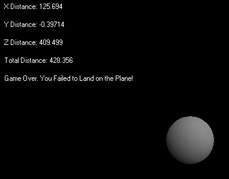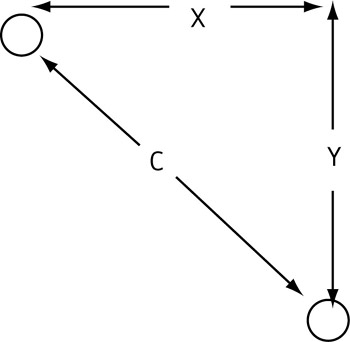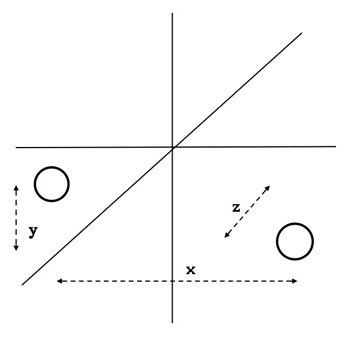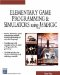# Sphere6

Here are the topics we will discuss in this section:

• Pythagorean Theorem in 3D

• Embedded if statements

• Inertia

• The SetGravity command

• Flags

This will be our first real game, not just a demonstration program like the previous ones. When the game begins you will see a sphere in front of you and a flat plane surface in the distance, at an altitude that is below the sphere. The sphere is falling slowly, and you can move it forward, backward, and to the sides. The goal is to position the sphere over the plane so that it lands on the plane, before the sphere falls too far. Figure 4.8 shows this game being run.Figure 4.8: Screenshot of Sphere6 game. The sphere must be maneuvered onto the landing surface before the sphere falls too far.

One of the first things we will change in our program is the way in which the numbers are displayed. You may recall from the previous program that the x distance was displayed with black letters in a somewhat tacky-looking white background. We will now get a much more professional looking display by making a black background (which will blend perfectly with the screen color) and by adding the following lines to the New StaticText statement:

` mytext = New StaticText(CurWindow," ",10,10,150,20); mytext.SetBackColor(GetRGB(0,0,0)); mytext.SetColor(GetRGB(255,255,255)); `

You will recognize the first line as the command that makes the text. The second line sets the background color of the text to black (RGB(0,0,0)). The third line sets the color of the letters in the text to white.

### The SetGravity Command

Here is the statement that will simulate gravity in our code:

` mysphere.SetGravity(0.002); `

This line makes mysphere fall towards the bottom of the screen at a rate determined by the number inside the parentheses. If you want to make mysphere fall more quickly, simply increase the number. By using negative numbers inside the parentheses you can make the mysphere fall up! For our game, 0.002 will be just about right.

mysphere will fall until it collides with a static object, so we must make sure we have enabled collision detection.

Another new feature of this program is that the camera will be made to follow behind the moving sphere at a set distance by using the following command:

` ocamera.Follow(mysphere,3000); `

This line makes the camera follow 3000 units behind mysphere . If we had used 3000 without the minus sign, the camera would have followed 3000 ahead of mysphere (and then it would actually be leading, not following!).

There is quite a bit of flexibility to the Follow statement that we are not yet using. This will be covered in the Flight Simulator project.

### Inertia

In this game, we will be concerned with inertia. Inertia is the tendency of an object to remain either at rest or in motion unless some force acts on it. For example, a baseball flies along its path until the bat exerts a force on it, and a car keeps moving until the brakes are applied. For the purpose of our game, we want our sphere to remain in motion until we tell it to stop. We also want it to change directions if we command it to do so. You may recall that in the previous programs, the sphere moved only for a few seconds when the arrow keys were pressed, and then stopped .

We will include inertia in our game by changing our Move statements. The first such change can be seen in the following if statement, which moves the sphere forward when the up arrow key is pressed:

` if(Keyboard.IsKeyDown(Keyboard.UP)) {    mysphere.Move(5000,50);  } `

In this case, mysphere will move a distance of 5000 units forward, and it will do so in 50 seconds. By using a large time (like 50), we ensure that mysphere continues moving for a while. The remaining arrow keys are programmed to move to the sides and back in the same way as described in earlier programs. When one of the other arrow keys are pressed, the new command is activated and the previous Move command is stopped.

For example, the user presses the up arrow key, which causes the sphere to move forward. A few seconds later, the user decides that the sphere needs to move to the right, so he presses the right arrow key. Forward motion then stops and the ball moves to the right.

We also need a way to stop the sphere when it is in motion, so in the following code, we tell the computer that when we press the letter S on the keyboard, it should stop the sphere. Of course, we could use any other available key for this, but S for Stop is easy to remember.

` if(Keyboard.IsKeyDown("S")) {   mysphere.Stop();  } `

Recall that we will be following behind the sphere with our camera, so it will be easy to tell if the sphere is properly lined up with the plane in the x direction. However, it won t be so easy to tell when the sphere is over the plane since you are viewing the sphere from behind, not from above. Being able to see this would be like kicking a ball and being able to see it move toward the goal from the same position from which you kicked it ”it s easy to tell that the ball is going between the goal posts, but it is impossible to tell exactly when the ball crosses the goal-line. Someone observing from above would be able to tell, but from the kicker s vantage point, it s not possible to know immediately.

We could solve this problem by presenting two views of the sphere: one from above and one from behind. We will do this in a later section. For now, we will simply calculate the distance from the sphere to the plane in the x, y, and z directions and present these data on the screen. This way, the user of the game will know when he has positioned the sphere over the plane.

Here are the commands we will use to determine the position of the sphere:

` distx = (mysphere.GetX) ; mytext.SetText("X Distance: " + distx); `

This should look familiar to you; these lines appeared in the previous program. The first line finds the x coordinate of mysphere , and the second line sets the text called mytext so it reads X Distance: , followed by the value of the x coordinate of mysphere . The x coordinate of the sphere is the same as the distance from the plane since we will be placing the plane at the origin (0,0,0).

In the next four lines, we find the y and z coordinates of the sphere and send them to the screen:

` disty = (mysphere.GetY) ; mytext2.SetText("Y Distance: " + disty); distz = (mysphere.GetZ) ; mytext3.SetText("Z Distance: " + distz); `

### The Pythagorean Theorem

To make our program work correctly, we would like to know the total distance between the sphere and the plane. Let s say we are dealing in two dimensions and we know how far apart two points are in the x and y directions. Take a look at Figure 4.9. In this figure, you can see that if X is the distance between the points in the x direction, and Y is the distance between them in the y direction, then we can find the distance C using the Pythagorean theorem, which is shown here:

` C = Sqrt(X * X + Y * Y) `Figure 4.9: The Pythagorean theorem for finding the distance C between two points.

Sqrt means the square root of whatever is inside the parentheses. So in this equation, we are squaring length X and adding it to the square of length Y, and then we are taking the square root of the whole thing.

The Pythagorean theorem can be extended to 3D by including the distance Z between the points, as is shown in Figure 4.10. The distance between two points in 3D space is represented as follows :

` C = Sqrt(X*X + Y*Y + Z*Z). `Figure 4.10: Distance between points in 3D space.

In other words, if we apply the 3D Pythagorean theorem to our situation, the distance between our sphere s center and the plane (at 0,0,0) can be represented as

` totaldist = Sqrt(distx*distx + disty*disty + distz*distz). `

Just for review, remember that the multiplication is done first and then the addition.

We will display this distance that results from this equation in a text box:

` mytext4.SetText("Total Distance: " + totaldist); `

You will probably recall being introduced to the docollision function. This function will come into play now because in order for the game to be won, the user will need to land his sphere on the plane ”in other words, he will collide the sphere and the plane.

We want several things to happen when the collision takes place: we want the current music to stop and a sound called osound2 to play, and we want to let the user know that he has achieved his goal by displaying a message on his screen. These actions are shown here:

` Function docollision {    omusic.Stop();     osound2.Play();    mytext5.SetText("Congratulations! You landed on      target!");     // all on one line } `

This seems logical enough when you look at it. However, if you were to try it you would find that the sound osound2 never plays in its entirety, causing an unpleasant result. In fact, it keeps playing only its very beginning over and over and over. Basically, if osound2 consisted of the song Jingle Bells the code would play Jingle ” Jingle ” Jingle ” Jingle ” repeatedly.

The reason this is happening is that the collision is constantly being detected , even after it has already occurred. If statements like omusic.Stop and the SetText command are repeated in the function, there is no problem because when these statements are repeated over and over, the user doesn t notice. However, with the osound2.Play() command, it is quite a different story. We only need this command to run once, and we next explain how to do this.

### Flags and Counters

Consider the following lines of code:

` counter = 0 while(1) //Beginning of game loop {  counter = counter + 1 if (counter == 1)  {     mytext.SetText(counter)  } // End of game loop } `

Let s figure out what is going on here. As you know, the program is constantly looping through the game loop. In the first loop, the variable counter starts out with a value of zero, but this is incremented by one when the program reaches the first line of the loop.

Then, at the if statement, the program decides whether to perform whatever is between the brackets. The first time the loop gets to the if statement, counter = 1, so the text displays 1.

The loop continues in this manner until the user exits the program, so the statement: counter = counter +1 is reached one more time. This time the counter is set to 2, and as a result, the if statement condition is not met. At this point, the program does not print mytext again, and it skips over whatever is inside the brackets contained by the if statement.

Now examine the code below, which contains a different version of the function docollision . If you understood the explanation above, you should have little trouble understanding how we now get the sound osound2 to play only once. Please note that we chose our variable name as flag instead of counter to illustrate that almost any name could be used for the variable that counts the number of loops :

` Function docollision {    flag = flag + 1;    omusic.Stop();    if(flag==1)      {        osound2.Play();      }    mytext5.SetText("Congratulations! You landed on     target!");// all on one line } `

Recall from the last section that two equal signs (==) are used to test values.

### Determining When the Game Is Lost or Won: Embedded if Statements

Now that we have seen what happens when the user successfully lands his sphere, we need to know when the user of the program fails to meet his objective. This happens when the sphere fails to land on and falls lower than the plane. The main way that we would know that the sphere had missed the plane would be if the y coordinate of the sphere ( disty ) fell below the level of the plane, which is at y = 0. So, if disty is less than or equal to 0 (<= 0), then the user has failed to land the sphere on the plane because the sphere has fallen too far.

If you are really astute, you might ask, But what about when the sphere and plane collide? Doesn t disty = 0 , make it seem as if the game has been lost when it hasn t? The answer is that disty is the distance to the sphere s center, so when the sphere and plane collide disty will have a value of about 50 (the radius of the sphere), unless the sphere hits the edge of the plane.

All that being said, we can account for the sphere missing its target by the following code:

` if(disty <= 0) { flag = flag+1; mysphere.SetGravity(0); mysphere.Stop(); omusic.Stop();   if(flag ==1)     {       osound.Play();     } mytext5.SetText("Game Over. You failed to land on the plane!");// all on one line } `

The first line expresses something new with the <= symbol; this line means If disty s value is less than or equal to zero. Other possible forms that use variations of the greater than/less than symbols and the equal sign include the following: if (x > = 20) , which means If x is greater than or equal to 20, and if (x < 25) , which means If x is less than 25.

You can see that by the use of flag in this code that we are implementing a similar scheme as we did with the docollision example earlier. This is set up so that the sound plays only once. Also noteworthy is the fact that there is one if statement within another. This is known as an embedded if statement .

### Running the Program

When you run the program, you will see the x , y, and z distances from the sphere to the plane displayed on your screen. You must adjust the z distance using the up and down arrow keys until you get the sphere closer than 100 units from the plane. Because the sphere is 100 units wide, there is no reason to get it any closer than that ”your sphere will collide with the plane as long as its distance from the plane is less than 100. Of course, you will also need to adjust the position of the sphere in the x direction, but this is done visually very easily and as a result, you really do not need the text message giving you the x distance.

 Note Remember from earlier discussions that pressing the S key stops the motion of the sphere (except under the influence of the gravity command).

Please type in the following code. Don t forget to import any sounds and music before you run it. This game uses the previous catch and melody , as well as a very nice sound called jump from the Rolling Ball 3D game in the Advanced Examples section.

### Sphere6 Code

` // Sphere6: 6th Spherelander game. Will try to land sphere //at plane center under the influence of gravity. We will // learn: // (a) set gravity // (b) camera follow command // (c) partially accounting for inertia // (d) calculating distance between objects - 3D //     PythagoreanTheorem // (e) embedded if statements // Create a new world with a new camera. oworld = New World(); ocamera = New Camera(oworld); //Initialize variables flag = 0; // Music omusic = New Music("melody"); omusic.SetLoop(ON,3); omusic.Play(); //Text message mytext = New StaticText(CurWindow," ",10,10,150,20); mytext.SetBackColor(GetRGB(0,0,0)); mytext.SetColor(GetRGB(255,255,255)); mytext2 = New StaticText(CurWindow," ",10,40,150,20); mytext2.SetBackColor(GetRGB(0,0,0)); mytext2.SetColor(GetRGB(255,255,255)); mytext3 = New StaticText(CurWindow," ",10,70,150,20); mytext3.SetBackColor(GetRGB(0,0,0)); mytext3.SetColor(GetRGB(255,255,255)); mytext4 = New StaticText(CurWindow," ",10,100,150,20); mytext4.SetBackColor(GetRGB(0,0,0)); mytext4.SetColor(GetRGB(255,255,255)); mytext5 = New StaticText(CurWindow," ",10,130,220,20); mytext5.SetBackColor(GetRGB(0,0,0)); mytext5.SetColor(GetRGB(255,255,255)); // Sounds osound = New Sound("catch"); osound.SetLoop(ON,1); osound2 = New Sound("jump"); // Create a sphere with Gouraud shading. mysphere = oworld.CreateSphere(100,100,100,20,20); //mysphere.SetPosition(100,500,1000); mysphere.SetPosition(500,500,1000); omaterial = New Material     (oworld,GetRGB(50,150,200),"lightblue");//one line omaterial.SetFlat(ON); mysphere.SetGouraud(ON); mysphere.ReplaceMaterial(omaterial); // Create a plane and rotate it so it's flat. myplane = oworld.CreatePlane(100,100); //myplane.SetPosition(0,200,1000); myplane.SetPosition(0,0,0); matp =New Material(oworld,GetRGB(0,100,50)); matp.SetFlat(ON); myplane.ReplaceMaterial(matp); myplane.SetAngle(-Pi/2,0,0); myplane.SetStatic(); // Camera oworld.Optimize(ocamera); ocamera.Follow(mysphere,3000); //Set collision detection. oworld.OnCollide = docollision; mysphere.SetCollision(TRUE,Object.COLLISION_TYPE_STOP); // Set gravity on. mysphere.SetGravity(0.002); //Game loop While(1) { if(Keyboard.IsKeyDown(Keyboard.UP))  {    mysphere.Move(5000,50);  } if(Keyboard.IsKeyDown(Keyboard.DOWN))  {    mysphere.Move(5000,50);  } if(Keyboard.IsKeyDown(Keyboard.RIGHT))  {    mysphere.MoveRight(5000,50);  } if(Keyboard.IsKeyDown(Keyboard.LEFT))  {    mysphere.MoveLeft(5000,50);  } // Distance calculations  distx = (mysphere.GetX) ;  mytext.SetText("X Distance: " + distx);  disty = (mysphere.GetY) ;  mytext2.SetText("Y Distance: " + disty);  distz = (mysphere.GetZ) ;  mytext3.SetText("Z Distance: " + distz);  totaldist = Sqrt(distx*distx + disty*disty +      distz*distz);//one line  mytext4.SetText("Total Distance: " + totaldist);  if(Keyboard.IsKeyDown("S"))   {     mysphere.Stop();   }  if(disty <= 0)  {  flag = flag+1;  mysphere.SetGravity(0.002);  mysphere.Stop();  omusic.Stop();  if(flag ==1)  {   osound.Play();  }  mytext5.SetText("Game Over. You Failed to Land on the  Plane!");//all on one line } //End of game loop follows. } Function docollision {      flag = flag + 1;     omusic.Stop();     if(flag==1)      {          osound2.Play();      }    mytext5.SetText("Congratulations! You landed on     target!");//all on one line `Elementary Game Programming & Simulations Using Jamagic (Charles River Media Game Development)
ISBN: 1584502614
EAN: 2147483647
Year: 2002
Pages: 105
Authors: Sergio Perez